• WORK
• TOOLS
• TRAINING
• VIEWS
• CONTACT

Views > Excel charts > Tufte in excel - the slopegraph

Slopegraphs such as the beauty below—produced by Edward Tufte in his The Visual Display of Quantitative Information (page 158)—organize complex information for viewing patterns and trends. The graph shows—for selected countries—government receipts as a percentage of GDP for two years. It plots 1970 composition down the left and 1979 composition down the right. The position of the labels on the left or right, makes it easy to get the ranking for either 1970 or 1979. The slopes of the lines make it easy to compare countries across years, that is, which is up or down and by how much.

Many tutorials have been written on how to build Slopegraphs in Excel. Here are three examples: Jon Peltier, myOnlineTraininghub, and E90E50.

The method—in its simplest form—involves selecting the dataset and, on the Insert tab, clicking on the Insert Line or Area Chart > Line. The chart should look like this:

Next—while the chart is selected—click on the Switch Row/Column icon in the Data group under the Chart Design tab. The chart should look like this:

Decluttering the chart by deleting the legend, the gridlines, the chart title, adjusting the ranges of the vertical axis, removing the chart outline and deleting the vertical axis transforms the chart into this:

# TUFTE IN EXCEL - THE SLOPEGRAPH

TUFTE IN EXCEL - THE SLOPEGRAPH

So far so good. But here is where troubles start. Moving from the above raw graph to Tufte’s graph requires the cumbersome process of manually changing the color of each data series (i.e. country)—one by one. The same should be done for the data labels. First, you have to add the data labels and then change their position to left (for year 1970). Next, you will have to add the series name to each data label and change the separator to space instead of comma. Moreover, you don't have much control over the space between the numerical value and the country name, and the order in which they are displayed. To make things worse, when a different dataset—with more or less data—is used some of the formatting done to the chart is lost and has to be redone again. Not efficient. Not fun.

To solve this problem—without resorting to VBA—I took advantage of named Excel Tables and the new Dynamic Arrays features of Excel (Only available in Office 365).

Tables allow the data source to expand and shrink based on different datasets without the need to adjust references and formulas. Dynamic Arrays allow for splitting the data series into 4 different data series:
1- One for the countries that went up (say in light blue color).
2- One for the countries that went down (say grey color)
3- One for the country with the steepest slope up (red color)
4- One for the country with the steepest slope down (red color)

And finally, Dynamic Arrays also allowed for handling the positions of overlapping labels. This is how Tufte's chart looks like using my template:

Also based on the work of Edward Tufte, below is a slopegraph showing the five and twenty-year cancer survival rates.

Lets increase the data density. Below is a slopegraph showing traffic fatality rates for the 52 U.S. states. Note that 7 levels of overlapping labels are accommodated. Shall we call this graph the Frequency-Slopegraph?

In this graph I used a monospaced font Consolas instead of the Trebuchet MS used above. Equal width fonts bring order to the chart by unifying the width of the labels.

Lets stress-test the template. The graph below is showing the Corruption Perception Index for 198 countries and territories. Here, I've reached the limit of the template, that is, 9 levels of overlapping labels are accommodated. If ten or more labels are in proximity to each other, then the tenth label and above will overlap.

The template includes options that allows the user to control certain elements of the graph as per the explanations below:

Lets tackle each of the challenges one by one. I’ll start with the number of data series problem. As explained earlier, each country having its own data series is not efficient. Solving this problem requires using scatterplots instead, as per the process shown below.

Now the process of pivoting the data and inserting empty rows should be automated. To do that I need to find a transformation function that links the location of the item in the original table to its location in the new table. To simplify this transformation function, I decided to insert two empty rows after each country—instead of one as shown previously. So if the original dataset consists of 15 countries I need to use Dynamic Arrays to spill a sequence of 58 rows—2 * (2 * n – 1), making 29 different items with two rows each. Hence, from the sequence bn: 1..58 I can evaluate an, and from an I can get to n—the location of the item in the original dataset as per below graph.

The Excel formulas required to automate the process are shown below. Formulas 1 to 4 create the transformation function that links the 15-row dataset to the new 58-row dataset needed for the scatterplot. Formula 5 gets the right x-coordinate for each data point. The four formulas labeled 6 segregate the original data into the four data series I mentioned above—that is, Up, Down, Max and Min. Note that all these formulas are Dynamic Arrays and spill down as needed when the data source shrinks or expands.

My solution involves stacking labels horizontally while maintaining a minimum distance between the labels. The template can handle up to 9 overlapping labels. Anything above 10 will be lumped together and needs to be adjusted manually.

The first thing to do is to spill the labels and data horizontally and vertically from the original dataset as shown in steps (1) and (2) below. Next, evaluate the proximity matrix as shown in (3) below. In this case, it is a 15x15 matrix of ones and zeros. One is assigned if the distance between two labels is less than a certain limit—say epsilon defined by the user—and zero otherwise. Because the matrix is symmetrical—that is, if for instance Canada is overlapping with Italy, then obviously Italy is overlapping with Canada—hence all the matrix entries above the diagonal should be set to zero. Next, I sum up the rows and calculate the frequency of overlap for each label as shown in (4) below. For example, a value of zero means no overlap, a value of 3 means that the label is overlapping with 3 other labels. And finally, a number between 0 and 8 is assigned to each label as shown in (5) below. This number defines the number of times the label should be shifted left or right. For example, 2 means that the label should be shifted twice. Note that the data in section (5) depends on a feedback loop from the next iteration as shown in (7) below.

This process is iterative. The template includes 8 iterations for each side of the graph. That is, 8 for the left labels and 8 for the right labels. In the second iteration, any label that has zero overlap from the previous iteration—section (4)—will be removed from the set by assigning a value #N/A as shown in section (6) below. The process is repeated 8 times till identifying all overlaps.

The next challenge is handling overlapping labels. Edward Tufte’s solution orders and stacks different graphs on top of each other to avoid lines crashing into words and numbers.

Another solution is to stack the labels vertically as per Alberto Cairo’s graph below.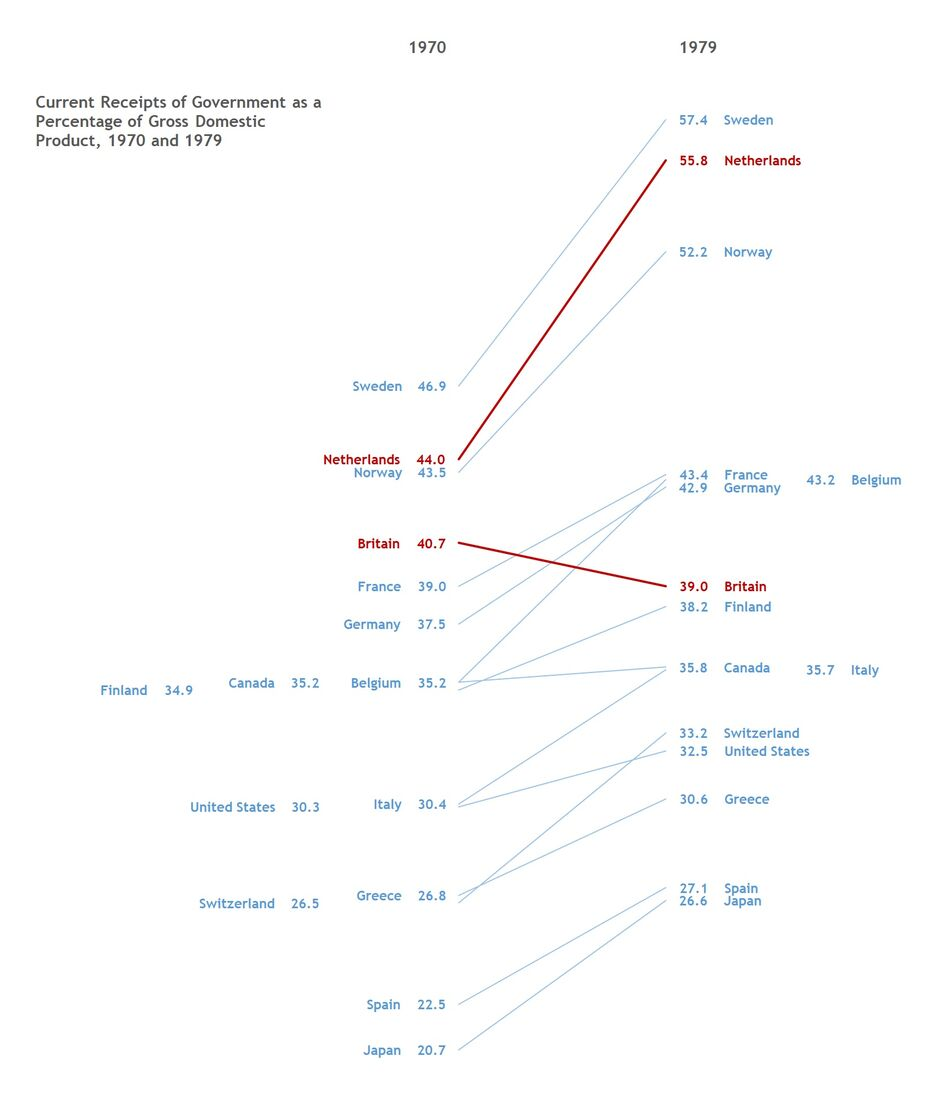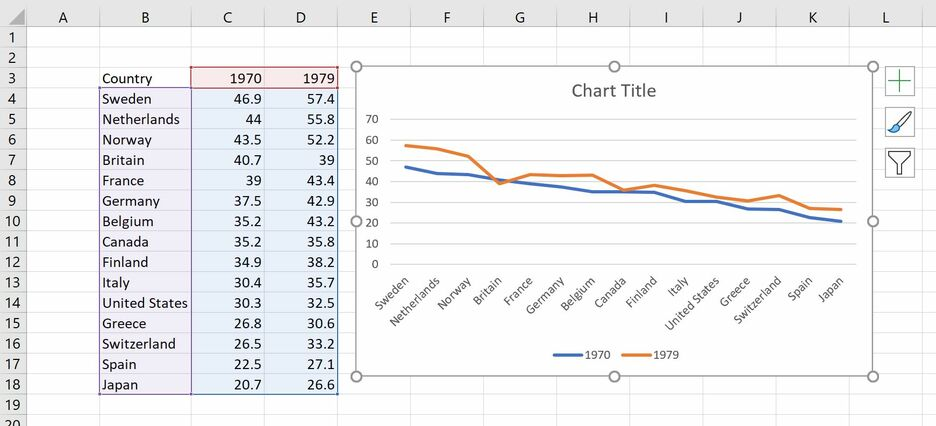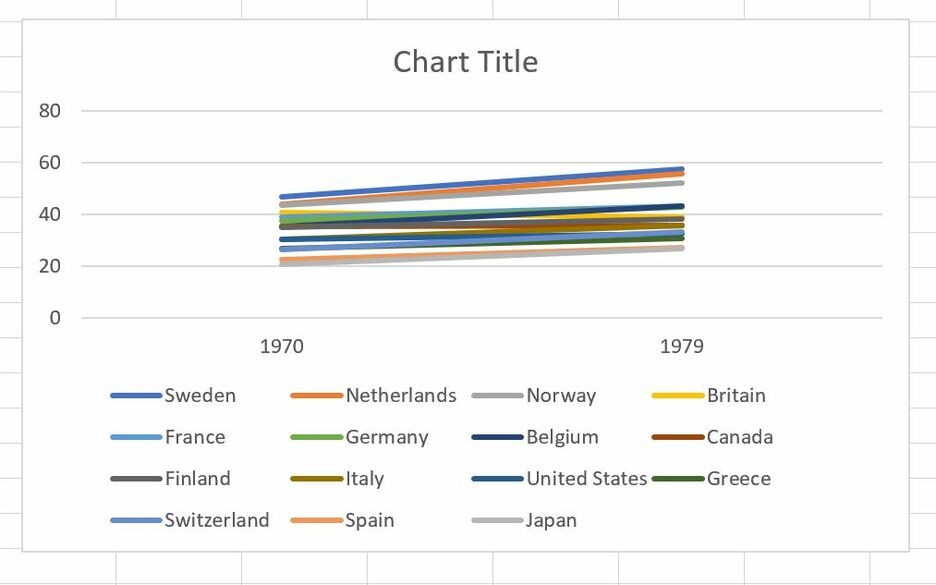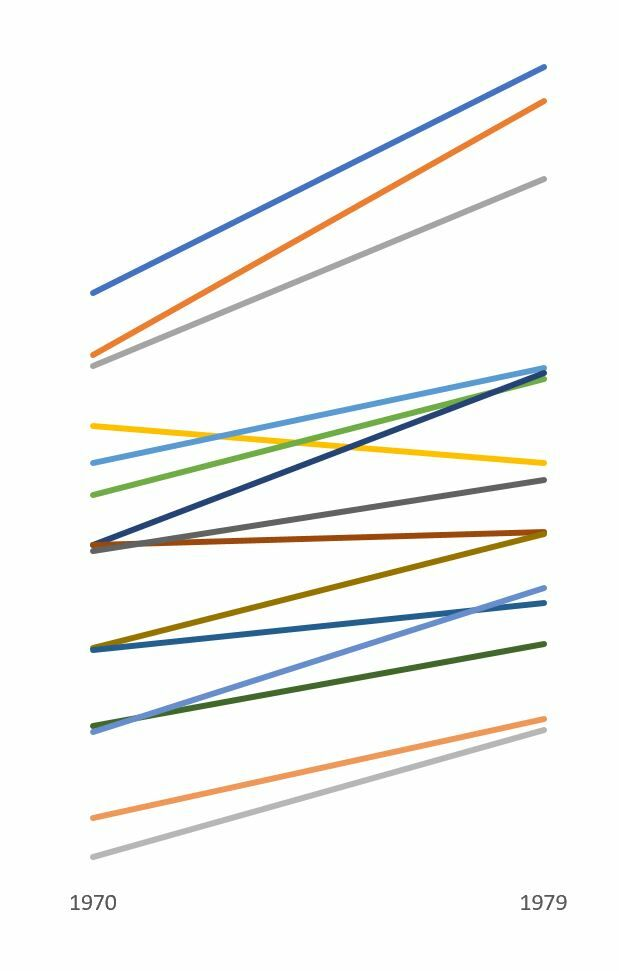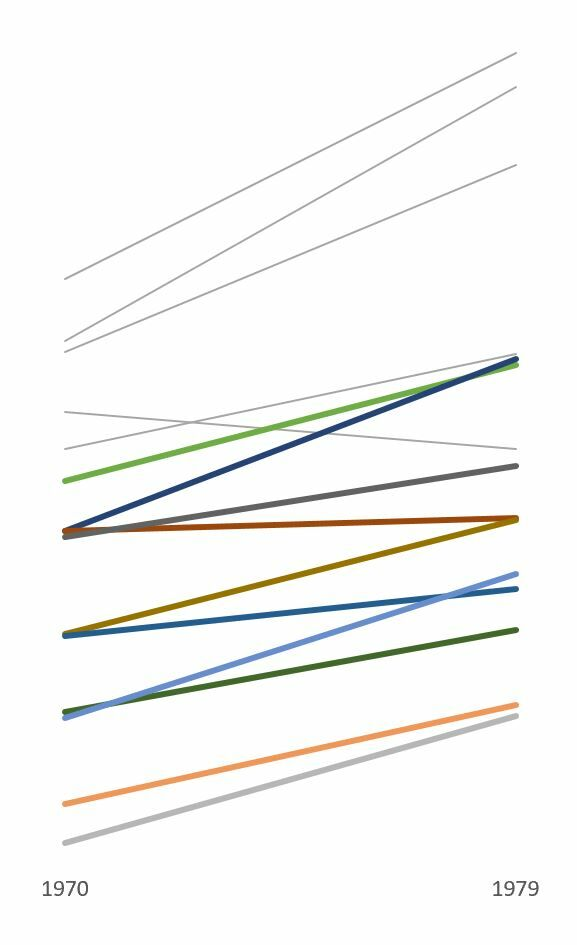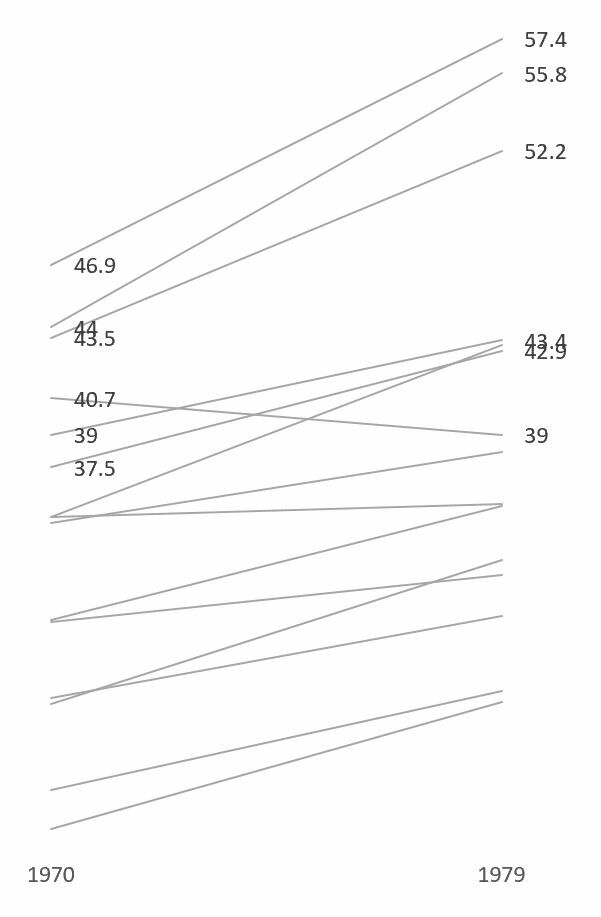1) Change color of data series—one by one.

2) Add data labels—one by one.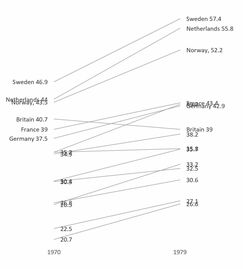3) Change label position, add series name and use space separator—one by one.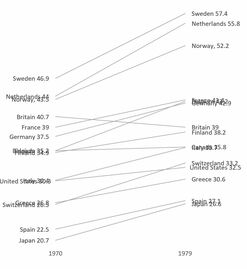4) Still, limited control over spacing between number and country, and their order.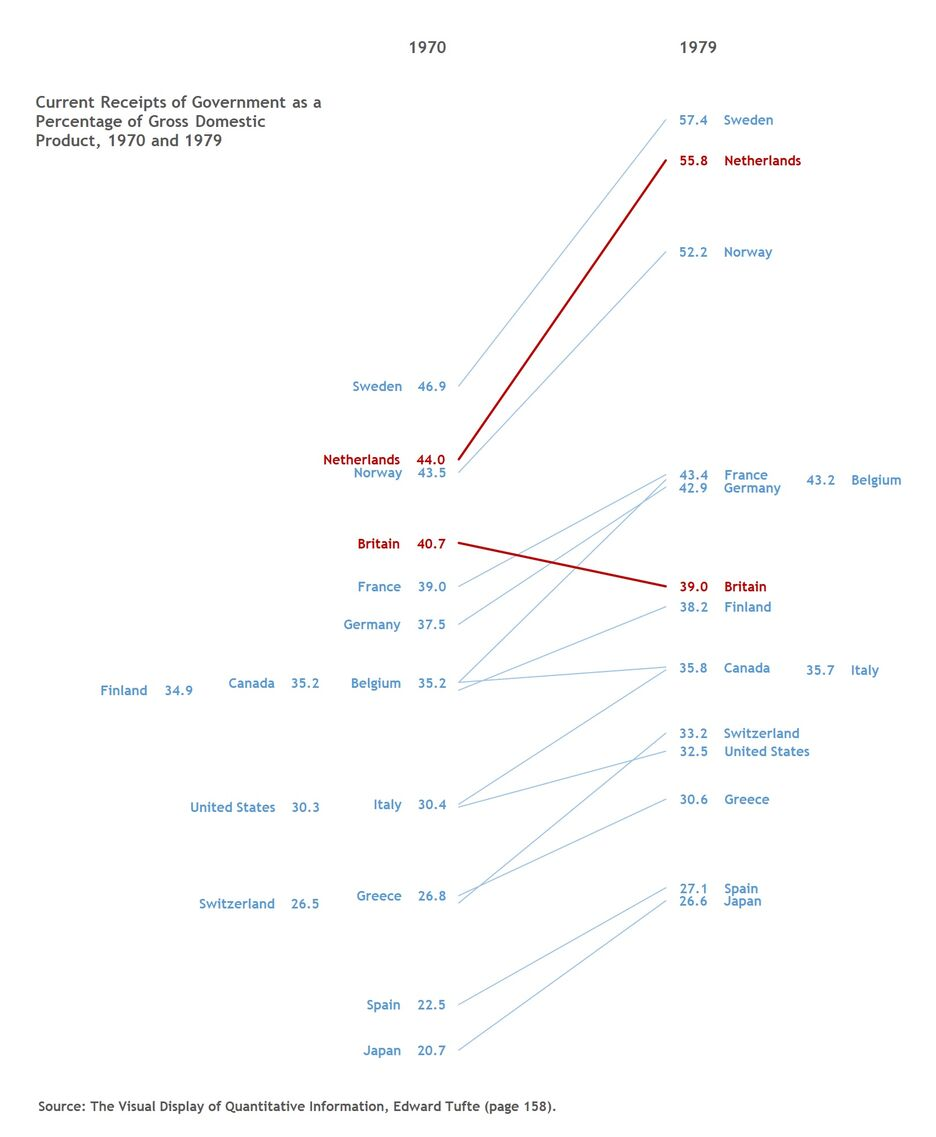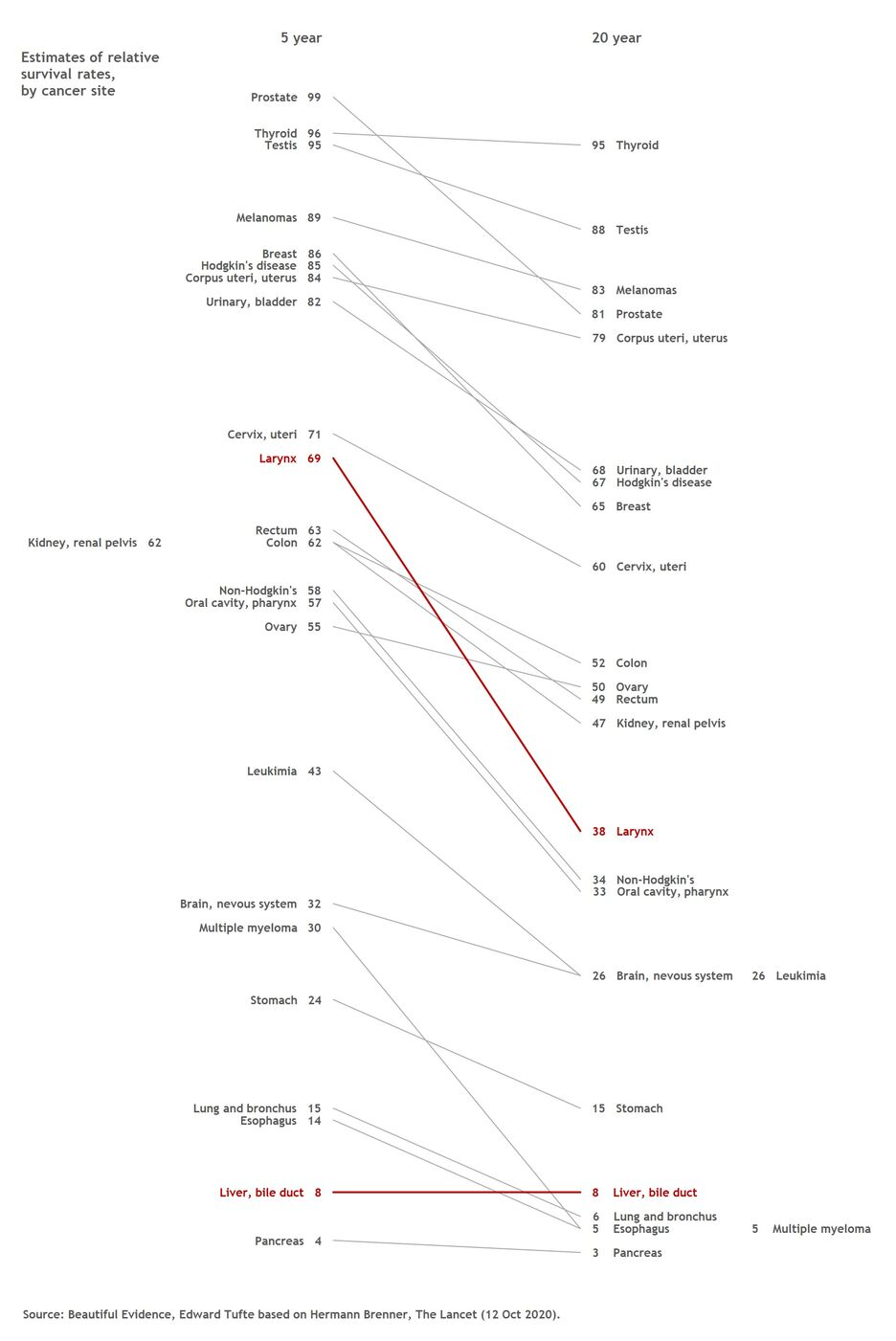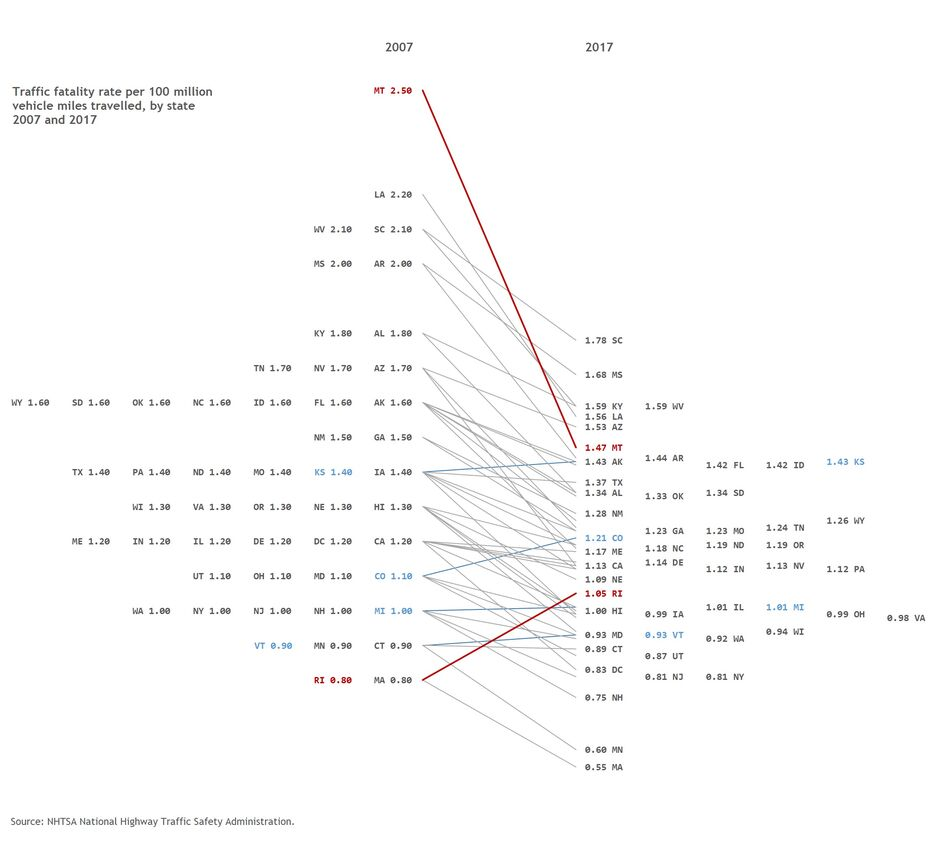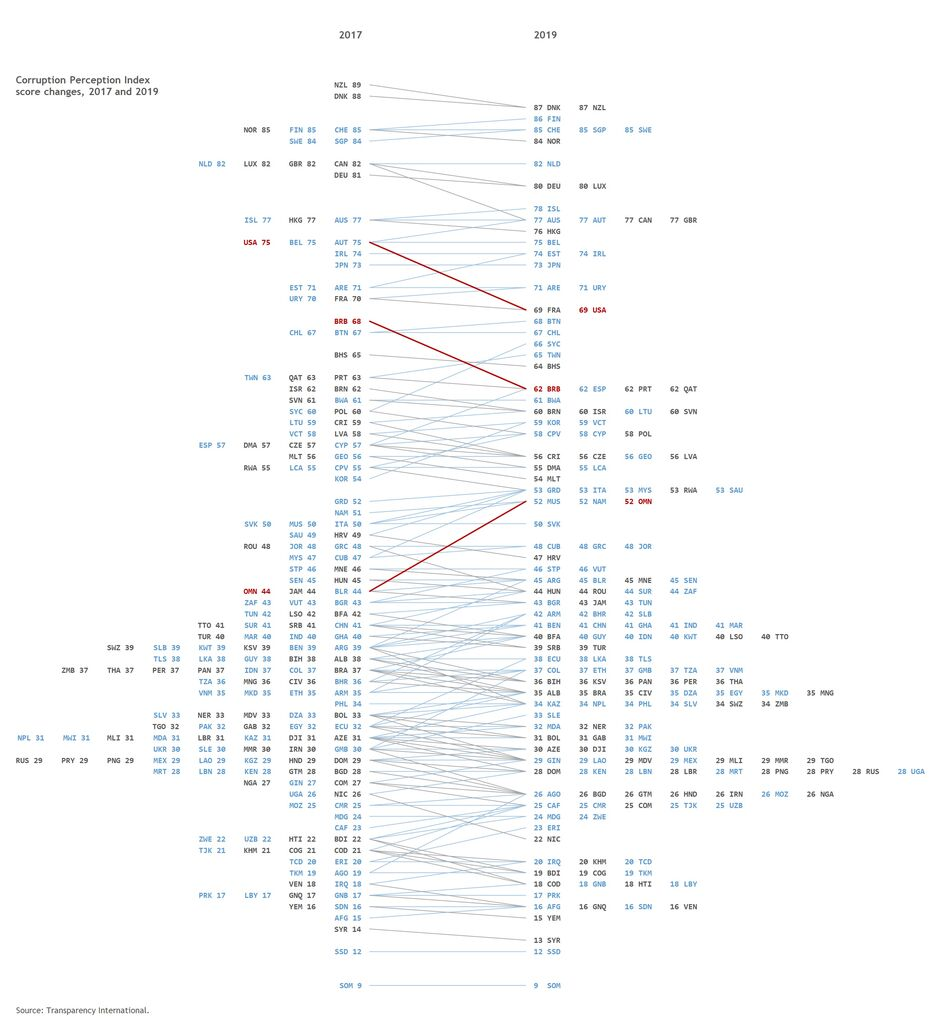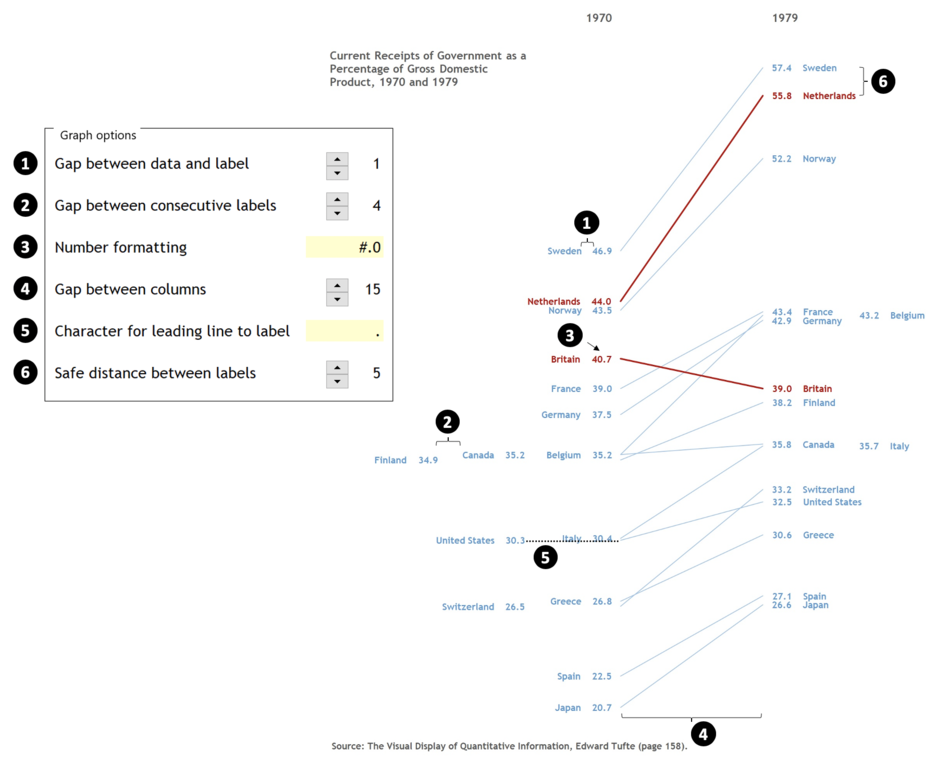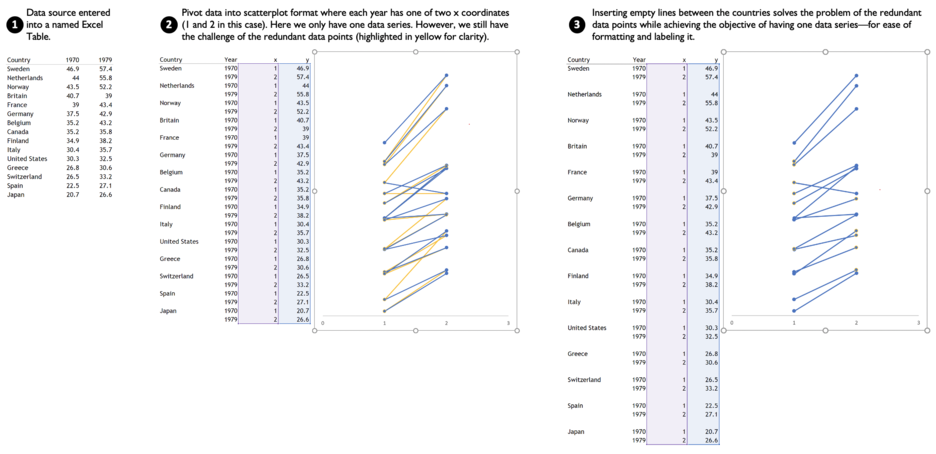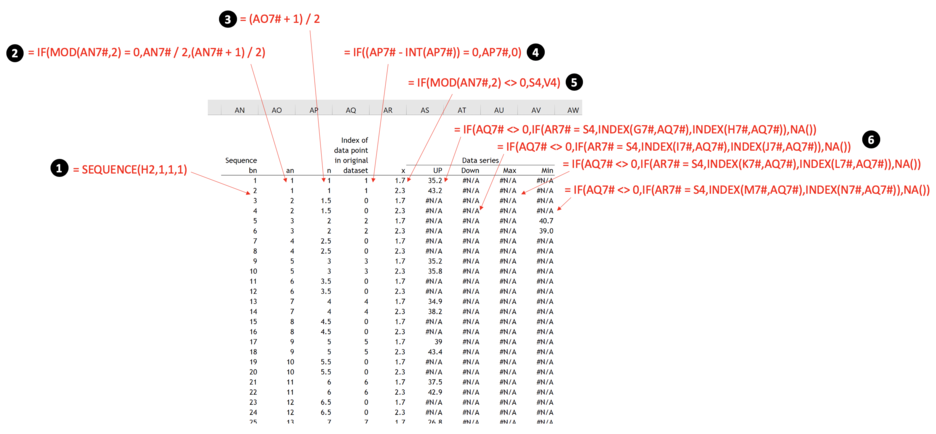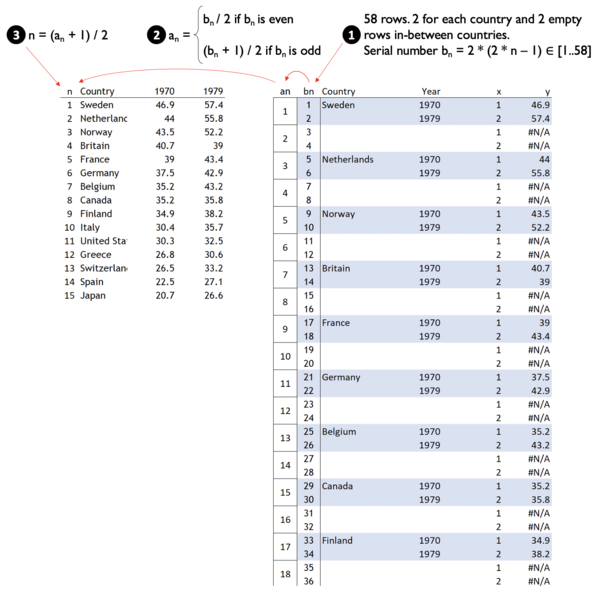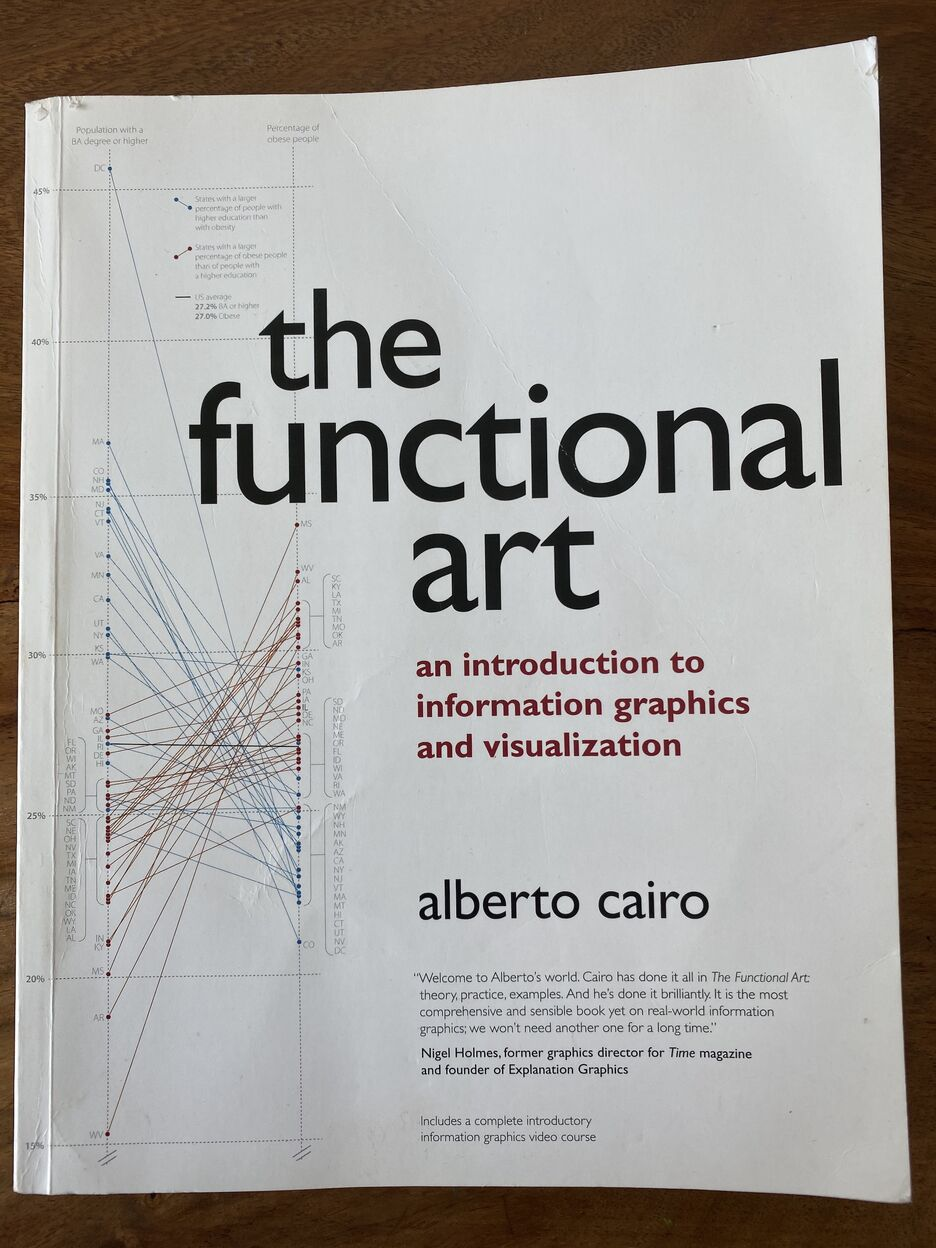File uses Dynamic Arrays available to Microsoft Office 365 subscribers.

Finally, the labels are adjusted with spaces (or any character specified by the user) according to the number of times they should be shifted left or right. Here I used the REPT function along with the numbers calculated in sections (5) and (7) as shown above.

Here is the formula I used for the labels on the left:

= IF(Y7# = 0,T7#,
IF(Y7# = 1,T7# & REPT(N3,Z7# + E4),
IF(Y7# = 2,T7# & REPT(N3,2 * (Z7# + E4)),
IF(Y7# = 3,T7# & REPT(N3,3 * (Z7# + E4)),
IF(Y7# = 4,T7# & REPT(N3,4 * (Z7# + E4)),
IF(Y7# = 5,T7# & REPT(N3,5 * (Z7# + E4)),
IF(Y7# = 6,T7# & REPT(N3,6 * (Z7# + E4)),
IF(Y7# = 7,T7# & REPT(N3,7 * (Z7# + E4)),
T7# & REPT(N3,8 * (Z7# + E4))))))))))

It looks complicated, but at its core it reads like this, where n is the number of times the label should be shifted left:

Original label & REPT(Character specified by the user, n * (Length of original label + Minimum space between labels))

Thank you for reading, and I hope you found this useful. If you have any questions, find me on Twitter and ask me anything.